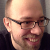# How to display objects as images in IPython

IPython has some neat functionality for displaying objects in ways that can be more informative than the standard `__repr__` representation. Both the IPython notebook and qtconsole support the display of png, jpeg and svg images. Furthermore, the IPython notebook can also display html, javascript, json and latex.

If you simply want to display an image you can achieve this using the `IPython.display.Image` class.

``````>>> from IPython.display import Image
>>> image = Image('tiny_tjelvar.png')
>>> image
``````

The last `image` call would result in the image below being displayed in the IPython qtconsole/notebook.However, suppose that you wanted to create an image representation of your own class. Let us illustrate this with the hypothetical example of an `ImageFile` class that simply stores the location of an image.

``````class ImageFile(object):
"""Class for storing an image location."""

def __init__(self, fpath):
self.fpath = fpath

def _repr_png_(self):
``````

The usage of the class above would be along the lines of the below.

``````>>> im_file = ImageFile('tiny_tjelvar.png')
>>> im_file
``````The example above would fall over if the file was not in png format. Let us make the code a little bit more robust by adding a naive file format check.

``````class ImageFile(object):
"""Class for storing an image location."""

def __init__(self, fpath):
self.fpath = fpath
self.format = fpath.split('.')[-1]

def _repr_png_(self):
if self.format == 'png':
``````

Finally, let us extend the class to be able to deal with jpeg and svg images as well.

``````class ImageFile(object):
"""Class for storing an image location."""

def __init__(self, fpath):
self.fpath = fpath
self.format = fpath.split('.')[-1]

def _repr_png_(self):
if self.format == 'png':